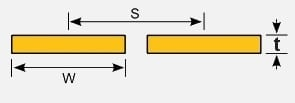Inductance

## Edge Coupled Trace Inductance Calculator### Inputs

Trace Width
w
Trace Separation
s
Trace Length
l
Trace Thickness
t
Relative Permeability
µr

### Output ⊕

Inductance:
3.75 x 10-8 H
Inductance per unit length:
7.50 x 10-7 H/m

View Notes

#### Calculation Notes

Coplanar traces are commonly found in printed circuit boards, where one trace is the signal and the other is the return. The trace separation should be constant along the trace length.

Factors that influence the inductance calculation include:-

• Trace width (w)
• Trace separation (s)
• Trace length (l)
• Trace thickness (t)
• Relative permeability (µr)

\mu_{0} = 4\pi \times 10^{-7}\;Wb\;A^{-1}\;m^{-1} (permeability of free space)

Inductance \approx l\times\frac{\mu_{0}\times \mu_{r}}{\pi}\times cosh^{-1}\;\frac{s}{w}

where

s \gg w, w > t

References:
• Electrical Circuit Theory and Technology (ISBN 978-1-85617-770-2)
• Signal Integrity Issues and Printed Circuit Board Design (ISBN 0-13-335947-6)

Disclaimer: The information and this tool are provided with no liability of any kind whatsoever, use at your own risk.